# The Two Amigos

I'd like to introduce Brett Shoelson as guest blogger for today. Many of you already know Brett from his work on the File Exchange Pick of the Week blog, or his posts on the comp.soft-sys.matlab newsgroup, or from his many presentations given on the road. I met Brett "virtually" many years ago, long before he came to work for MathWorks, through his posts on the newsgroup. Brett's been doing fun, interesting, and even useful things to images using MATLAB for quite a long time.

A few weeks ago Brett posted the Two Amigos Challenge on the Pick of the Week blog. Readers of the blog know that long-time contributor Bob Bemis (Hi, Bob!) has moved on from MathWorks and isn't posting there anymore. Brett challenged his readers to digitally retouch the "Three Amigos" picture on the blog and turn it into a "Two Amigos" picture using only MathWorks products.

I was so impressed with Brett's own solution to the Challenge that I invited him to show it to my readers here.

So here is Brett's solution to the Two Amigos Challenge ...

... in which we remove a subject from a photograph, and digitally retouch it to conceal the modification...

### Get/display image

url = 'https://blogs.mathworks.com/images/pick/threeamigos-800w.jpg';
togglefig('Original')
imshow(original);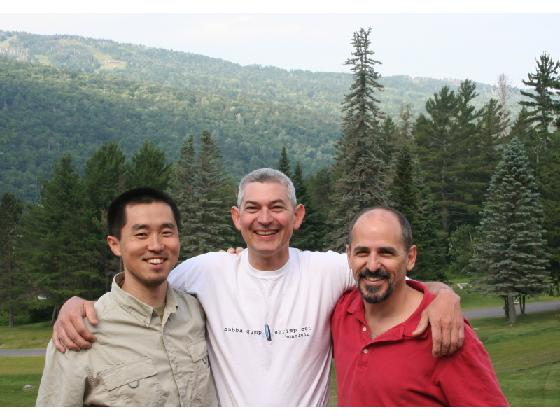NOTE: togglefig is a helper function I use often. If a figure with the specified title exists, activate and use it. Otherwise, create it.

My approach is going to start with creating a segmentation mask of Jiro, Bob, and Brett, and then creating individual segmentation masks for each.

### Decide on a color plane/colorspace

First, let's explore the information in the R,G, and B planes individually, as well as in the HSV, YCbCr, and LAB color spaces.

exploreRGB(original,'advanced')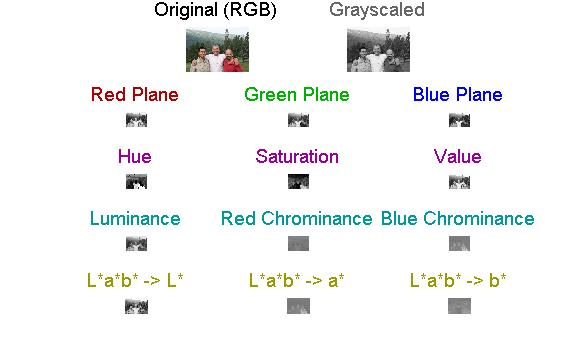### The Three Amigos

Let's segment the Three Amigos in the LAB colorspace

cform = makecform('srgb2lab');

% A first pass at noise reduction using a median filter, operating plane-by-plane:
for ii = 1:3
original(:,:,ii) = medfilt2(original(:,:,ii),[6 6]);
end
lab = applycform(original,cform);

% Now we're going to threshold the "a*" colorspace:
a = lab(:,:,2);
threeAmigos = im2bw(a,0.5);
togglefig('Three Amigos')
imshow(threeAmigos)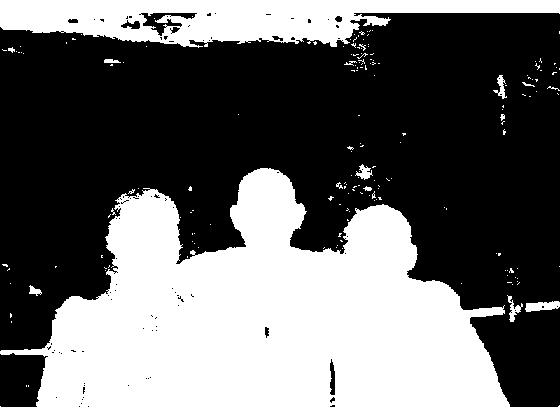### Now we can clean that up a bit

Fill holes...

threeAmigos = imfill(threeAmigos,'holes');
% ...calculate connected components...
cc = bwconncomp(threeAmigos);
% ...calculate the areas of all blobs in the image...
stats = regionprops(cc,'Area');
%...and keep only the largest:
A = [stats.Area];
[~,biggest] = max(A);
threeAmigos(labelmatrix(cc)~=biggest) = 0;
togglefig('Three Amigos')
imshow(threeAmigos)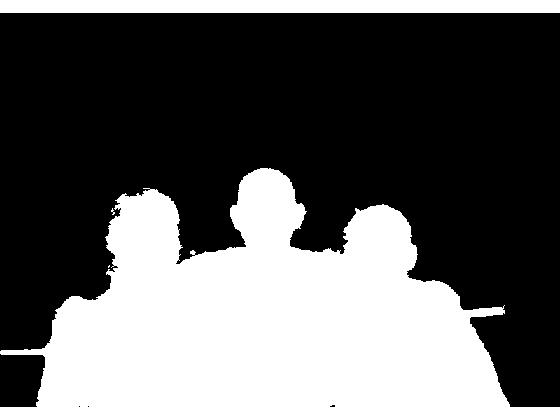### Oops...lost Jiro's hair!

That did a reasonable job of segmenting the Three Amigos. But I seem to have lost Jiro's hair, and he might want it back. Let's go back and find it....

jirosHair = original(:,:,3) <= 25.5;
% Get rid of "small" (defined here as <= 1050-pixel) blobs
jirosHair = bwareaopen(jirosHair,1050);
togglefig('Jiro''s Hair')
imshow(jirosHair)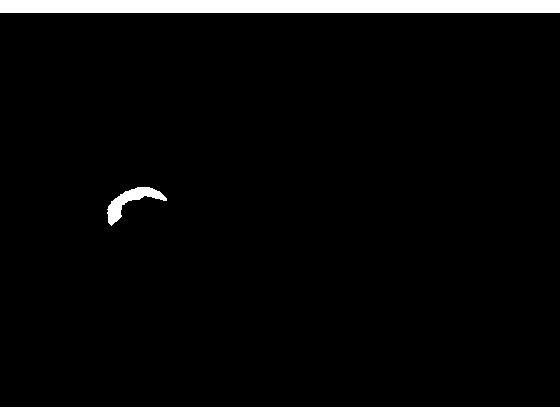Now we can reconstruct the Three Amigos, in all their hairy (or hairless) glory

% Binary OR:
threeAmigos = threeAmigos | jirosHair;
togglefig('Three Amigos')
imshow(threeAmigos)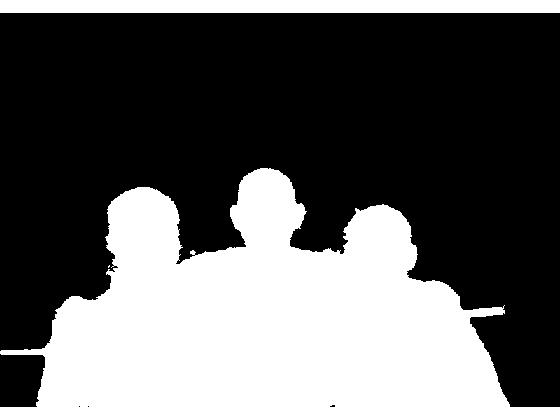### Get rid of the road and clean things up a bit

...by morphologically opening, then closing, the image:

threeAmigos = imopen(threeAmigos,strel('disk',19));
threeAmigos = imerode(threeAmigos,strel('disk',1));
togglefig('Three Amigos')
imshow(threeAmigos)### Three Amigos in color

Mask the background; here we set to zero all pixels outside of the threeAmigos mask using logical indexing:

amigosColor = original;
r = amigosColor(:,:,1);
r(~threeAmigos)= 0;
g = amigosColor(:,:,2);
g(~threeAmigos)= 0;
b = amigosColor(:,:,3);
b(~threeAmigos)= 0;
% Concatenate in the "3rd dimension" to re-constitute an RGB image.
amigosColor = cat(3,r,g,b);
togglefig('Amigos in Color')
imshow(amigosColor)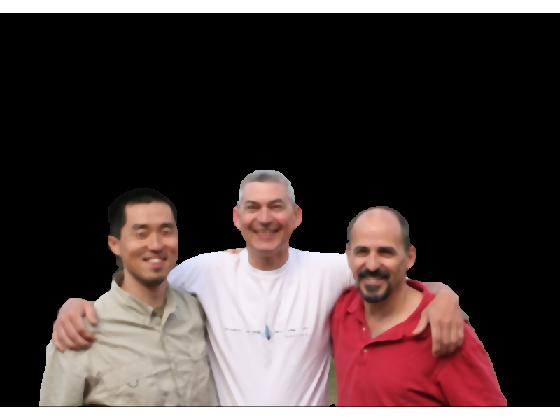Now we can concentrate on segmenting individual masks

### Three Amigos in pseudocolor

Let's exaggerate the differences to make this easier

amigosColor = decorrstretch(amigosColor);
togglefig('Amigos in Pseudocolor')
imshow(amigosColor)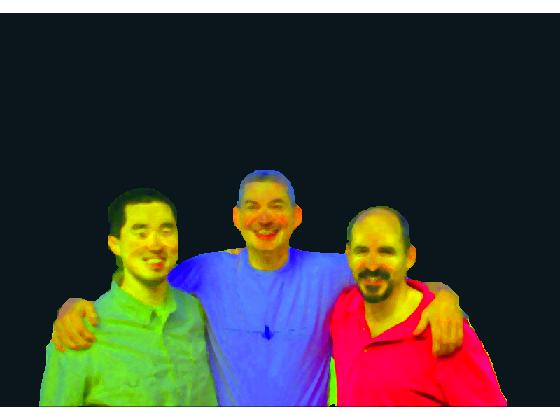### Again, look at some options for subsequent segmentation spaces

exploreRGB(amigosColor,'advanced');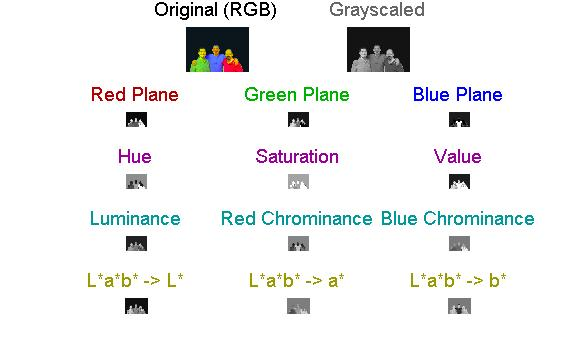### Finding Bob

Let's again work in the LAB colorspace

lab2 = applycform(amigosColor,cform);
% This time, we will use the "b* colorspace"
b2 = lab2(:,:,3);
% Segment Bob:
bobBodyHair = ~im2bw(b2,0.47);
bobBodyHair = imclose(bobBodyHair,strel('disk',3));
bobBodyHair = bwareaopen(bobBodyHair,50);

%Bob is in the middle; let's keep only the two "middle" regions (let's say,
%within 30 pixels of the center)
cc = bwconncomp(bobBodyHair);
stats = regionprops(cc,'Centroid');
C = reshape([stats.Centroid],2,[])';
inds = find(C(:,1) > size(bobBodyHair,2)/2 - 30 &  C(:,1) < size(bobBodyHair,2)/2 + 30);
bobBodyHair = ismember(labelmatrix(cc), inds);
togglefig('Finding Bob')
imshow(bobBodyHair)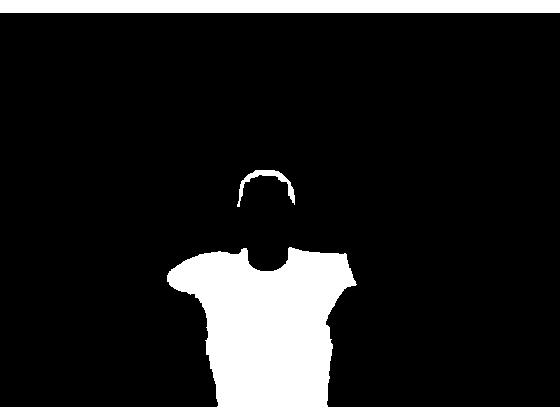### Now Bob's face...

Still working with "b*," but using a different threshold:

bobFace = im2bw(b2,0.56);
bobFace = imclearborder(bobFace);
cc = bwconncomp(bobFace);
stats = regionprops(cc,'Area');
A = [stats.Area];
[~,biggest] = max(A);
bobFace(labelmatrix(cc)~=biggest) = 0;
togglefig('Finding Bob')
imshow(bobFace)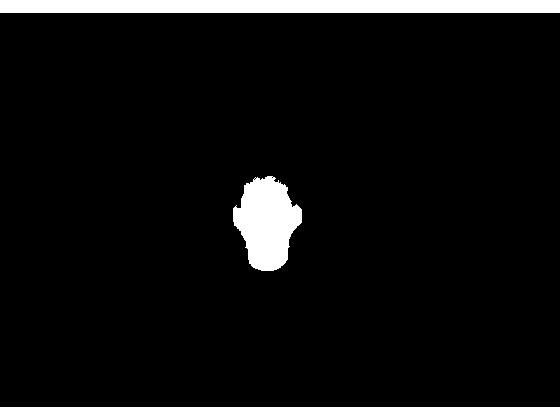### Reconstructing Bob

bob = bobBodyHair | bobFace;
bob = imfill(bob,'holes');
togglefig('Finding Bob')
imshow(bob)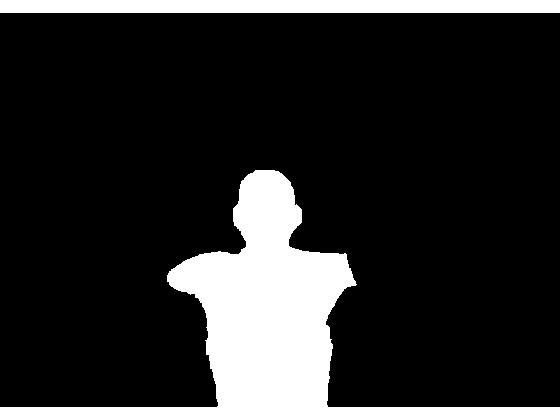### Jiro and Brett...

jiroBrett = threeAmigos & ~bob;
jiroBrett = bwareaopen(jiroBrett,300);
togglefig('Jiro and Brett')
imshow(jiroBrett)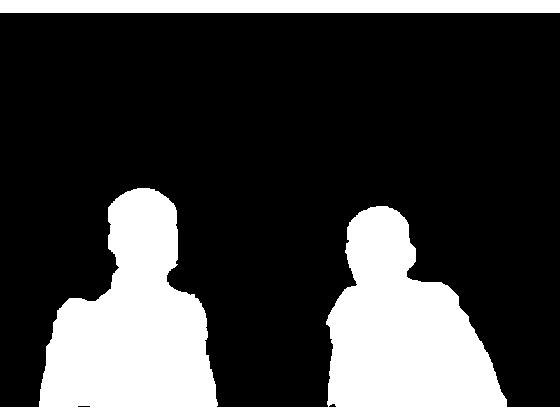### Jiro, and Brett

The connected components matrix is very convenient here:

cc = bwconncomp(jiroBrett);
jiro = labelmatrix(cc) == 1;
brett = labelmatrix(cc) == 2;

togglefig('Jiro')
imshow(jiro);
title('Jiro')
togglefig('Brett')
imshow(brett)
title('Brett')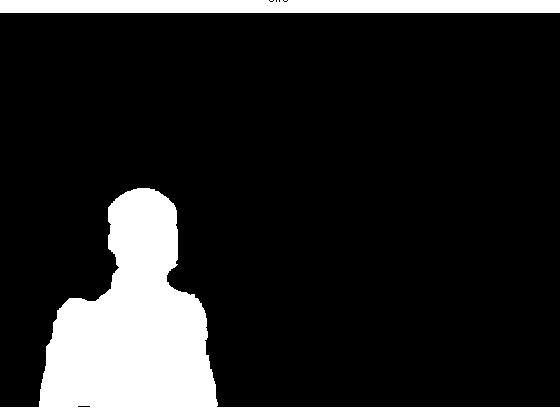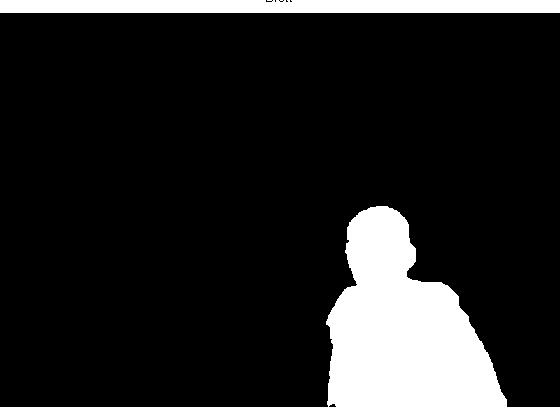### Now let's shift the rgb images to close the "Bob gap"

How "wide" is Bob at the bottom of the image? (Plus a bit of padding.)

shiftAmt = floor(nnz(bob(end,:))/2) + 7;

### Now we can move Jiro and Brett into the space Bob once occupied

twoAmigos = circshift(jiro,[0 shiftAmt]) | circshift(brett,[0,-shiftAmt]);
togglefig('Two Amigos');
imshow(twoAmigos);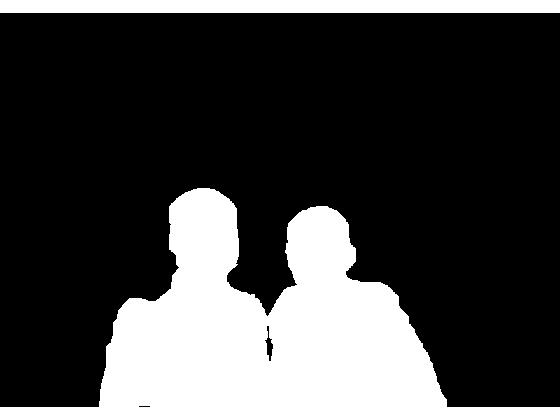### Two Amigos, in color

We can now create a color image that excludes Bob

% (We re-read the original because we reduced noise in it above using median
% filtering, prior to masking. Now we want the unsmoothed original back.)

rJiro = original(:,:,1);
rJiro(~jiro) = 0;
rJiro = circshift(rJiro,[0 shiftAmt]);
gJiro = original(:,:,2);
gJiro(~jiro) = 0;
gJiro = circshift(gJiro,[0 shiftAmt]);
bJiro = original(:,:,3);
bJiro(~jiro) = 0;
bJiro = circshift(bJiro,[0 shiftAmt]);

rBrett = original(:,:,1);
rBrett(~brett) = 0;
rBrett = circshift(rBrett,[0 -shiftAmt]);
gBrett = original(:,:,2);
gBrett(~brett) = 0;
gBrett = circshift(gBrett,[0 -shiftAmt]);
bBrett = original(:,:,3);
bBrett(~brett) = 0;
bBrett = circshift(bBrett,[0 -shiftAmt]);

r = max(rJiro,rBrett);
g = max(gJiro,gBrett);
b = max(bJiro,bBrett);
rgb = cat(3,r,g,b);
togglefig('Two Amigos Color')
imshow(rgb)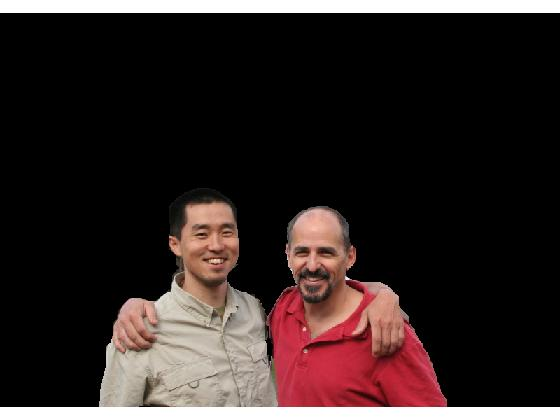### Add a background back in

Rather than try to "fix" the original background, I'm going to select a new backdrop for the "Two Amigos": ('forest.tif' is a sample image in the Image Processing Toolbox.)

[bg,map] = imread('forest.tif');
bg = ind2rgb(bg,map);
% Resize it...
bg = imresize(bg,size(r,1)/size(bg,1),'bicubic');
bg = bg(:,1:size(r,2),:);
bg = im2uint8(bg);
togglefig('(New) Background')
imshow(bg)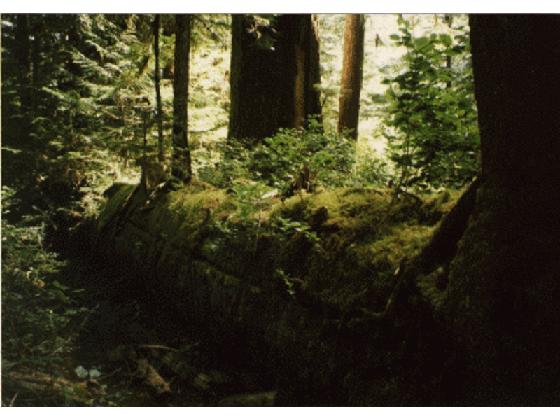### Finally, we drop Jiro and Brett into the new background

bgR = bg(:,:,1);
bgR(twoAmigos) = 0;
bgG = bg(:,:,2);
bgG(twoAmigos) = 0;
bgB = bg(:,:,3);
bgB(twoAmigos) = 0;
r = max(r,bgR);
g = max(g,bgG);
b = max(b,bgB);
rgb = cat(3,r,g,b);
togglefig('Two Amigos Color')
imshow(rgb)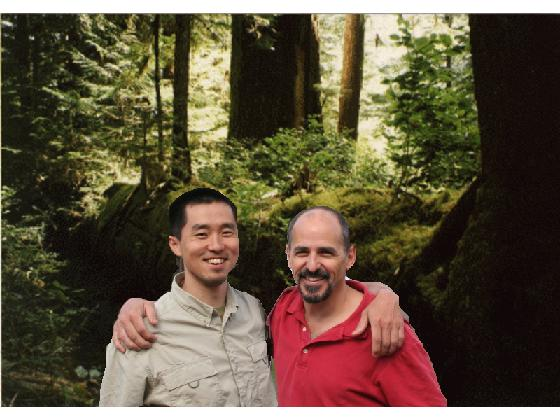### One more bit of cleanup:

The edge is a bit rough...

brettJiroEdge = bwperim(twoAmigos);
brettJiroEdge = imdilate(brettJiroEdge,strel('disk',9));
togglefig('Two Amigos Outline')
imshow(brettJiroEdge)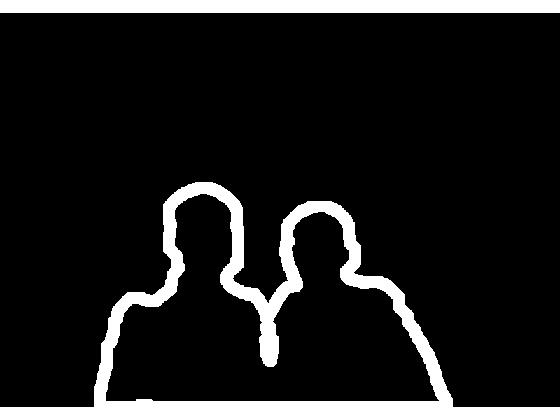### Final result

Soften the edges: we can soften those edges a bit by applying locally an averaging filter where the (dilated) outline is logically true:

H = fspecial('average');
% Roifilt2 is a 2-D filter. We can use it on RGB images, but we have to
% operate plane-by-plane. But first, it's good practice to preallocate the
% final matrix:
FINAL = zeros(size(rgb),'uint8');
for ii = 1:3
FINAL(:,:,ii) = roifilt2(H,rgb(:,:,ii),brettJiroEdge);
end
FINAL = imcrop(FINAL,[58 90 691 471]);
togglefig('Final Result')
imshow(FINAL)

% et voila!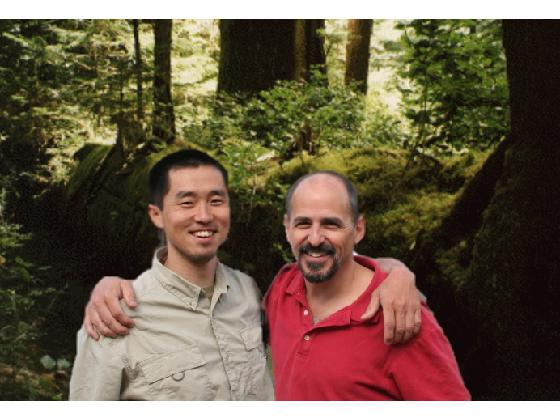Published with MATLAB® 7.11

|